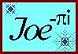Purlins following a Sloped Ridge Line
intercept plane of Main Roof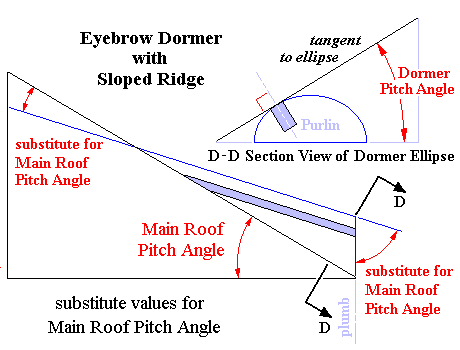Dormer with Sloped Ridge Line
Purlins following an Elliptic Arc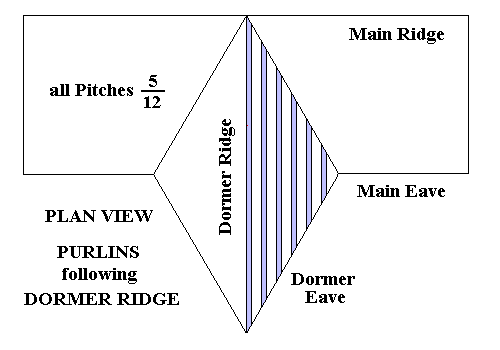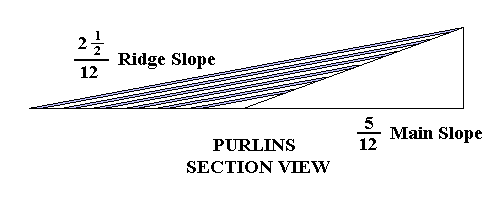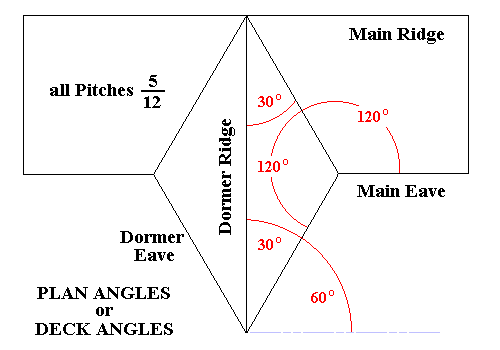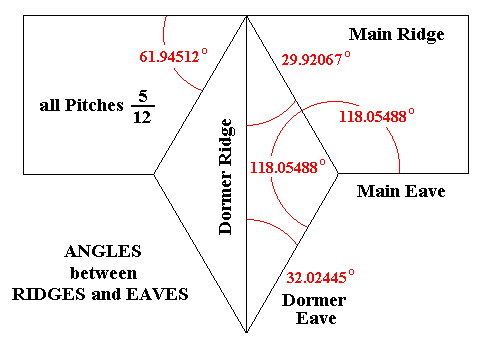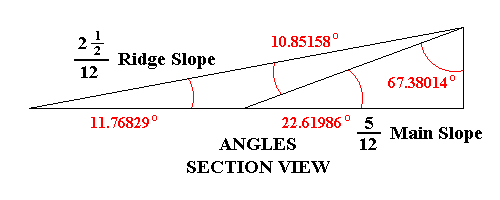Resolving the Principal Framing Angles

 NOTE : The following calculations may be validated independently on any scientific calculator with trig functions or a Construction Master calculator. GIVEN : All Common Rafter Pitches = 5 ÷ 12 Dormer Ridge Slope = 2 1/2 ÷ 12 as viewed in elevation Main Ridge and Dormer Ridge intercept = 90° as viewed in plan Solution of Plan Angles or Deck Angles The Dormer Ridge is equivalent to a Hip Rafter Ridge or Valley Rafter Trough Hip-Valley Pitch = Common Pitch × sin Deck Angle Therefore sin Deck Angle = Hip-Valley Pitch ÷ Common Pitch and Deck Angle = arcsin (Hip-Valley Pitch ÷ Common Pitch) = arcsin (2.5 ÷ 5) = arcsin (.5) = 30° The Total Deck Angle between the Dormer Eaves = 2 × 30° = 60° The Total Deck Angle between the Dormer Eave and the Main Eave = 240° Lag Calculator Entries : 5 ÷ 12 , 5 ÷ 12 , 60° Click on or button (equal Common Pitches; Main Side = Adjacent Side) SS = Common Pitch Angle = 22.61986° DD = Deck Angle or Plan Angle = 30.00000° R1 = Hip-Valley Pitch Angle = 11.76829° (= 2 1/2 ÷ 12 , as required) 90° - P2 = Angle on Roof Plane between Dormer Eave and Dormer Ridge = Sheathing Angle = 32.02445° C5 = Backing Angle for Dormer Ridge Line = 19.45623° Lag Calculator Entries : 5 ÷ 12 , 5 ÷ 12 , 240° Click on or button (equal Common Pitches; Main Side = Adjacent Side) SS = Common Pitch Angle = 22.61986° DD = Deck Angle or Plan Angle = 120.00000° R1 = Hip-Valley Pitch Angle = 19.84167° (= 4 11/32 ÷ 12) 90° - P2 = Angle on Roof Plane between Valley Trough and Main Ridge = Sheathing Angle = 61.94512° C5 = Backing Angle for Valley Trough Line = 11.08749° Angles on the Triangle of the Dormer Roof Plane : Angle on Roof Plane between Valley Trough and Main Eave = 180° - 61.94512° = 118.05488° Angle on Roof Plane between Valley Trough and Dormer Ridge = 180° - (118.05488° + 32.02445°) = Sheathing Angle = 29.92067°

Resolving the Compound Angles on the Dormer Purlins
Purlins intercept Main Roof Calculator

Purlins intercept slope of Main Roof
 Calculator Entries Select Pitch Angle mode Main Roof Rise = 10.85158° (Section View, angle of intercept with Main Roof) Dormer Rise = 19.45623° (the Backing Angle along the Dormer Ridge Line, therefore the Dormer Pitch Angle as viewed in section) Calculator Returns Face set in Dormer Roof Saw Blade Bevel = 67.82502° (complement of 2 × Backing Angle = Sleeper Angle = 2 × 11.08749° = 22.17498° = Dihedral Angle between the planes of the Dormer Roof and the 5/12 Main Roof) Angle on the Stick = 29.92068° (angle between Dormer Ridge and Valley Trough) Sheathing Angles on Main Roof Plane Sheathing Angle = 61.94511° (angle between Main Ridge and Valley Trough)

Purlins intercept level plane of Deck
 Calculator Entries Select Pitch Angle mode Main Roof Rise = 11.76829° (Section View, angle of intercept with Deck) Dormer Rise = 19.45623° (the Backing Angle along the Dormer Ridge Line, therefore the Dormer Pitch Angle as viewed in section) Calculator Returns Face set in Dormer Roof Saw Blade Bevel = 67.38014° (complement of the specified 5/12 Common Pitch Angle) Angle on the Stick = 32.02445° (angle measured on the plane of the roof between Dormer Ridge and Deck) Sheathing Angles on Main Roof Plane Sheathing Angle = 59.99999° (angle measured on the plane of the Deck, complement of the specified 30° Dormer Deck Angle)